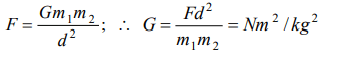## Units and Measurement Questions and Answers Part-1

1. Light year is a unit of
a) Time
b) Mass
c) Distance
d) Energy

Explanation: Light year is a distance which light travels in one year.

2. The magnitude of any physical quantity
a) Depends on the method of measurement
b) Does not depend on the method of measurement
c) Is more in SI system than in CGS system
d) Directly proportional to the fundamental units of mass, length and time

Explanation: Because magnitude is absolute

3.Which of the following is not equal to watt
a) Joule/second
b) $Ampere\times volt$
c) $\left(Ampere\right)^{2}\times ohm$
d) Ampere/volt

Explanation: Watt=Joule/second = Ampere×volt = Ampere2×Ohm

4. Newton–second is the unit of
a) Velocity
b) Angular momentum
c) Momentum
d) Energy

Explanation: Impulse = change in momentum = F × t
So the unit of momentum will be equal to Newton-sec.

5. Which of the following is not represented in correct unit
a) $\frac{Stress}{Strain}=N\diagup m^{2}$
b) Surface tension = N/m
c) $Energy=kg-m/sec$
d) $Pressure=N\diagup m^{2}$

Explanation: Unit of energy will be kg - m2 /sec2

6. One second is equal to
a) 1650763.73 time periods of Kr clock
b) 652189.63 time periods of Kr clock
c) 1650763.73 time periods of Cs clock
d) 9192631770 time periods of Cs clock

Explanation: It is by standard definition

7. One nanometre is equal to
a) $10^{9}mm$
b) $10^{-6}cm$
c)$10^{-7}cm$
d) $10^{-9}cm$

Explanation: 1 nm = 10-9m = 10-7cm

8. A micron is related to centimetre as
a) $1 micron=10^{-8}cm$
b)$1 micron=10^{-6}cm$
c) $1 micron=10^{-5}cm$
d) $1 micron=10^{-4}cm$

Explanation: 1 micron = 10-6m = 10-4cm

9. The unit of power is
a) Joule
b) Joule per second only
c) Joule per second and watt both
d) Only watt

a) $kg-m sec^{-1}$
b) $N m^{-1}sec$
c) $N m ^{2}K g ^{-2}$
d) $kg m sec^{-1}$